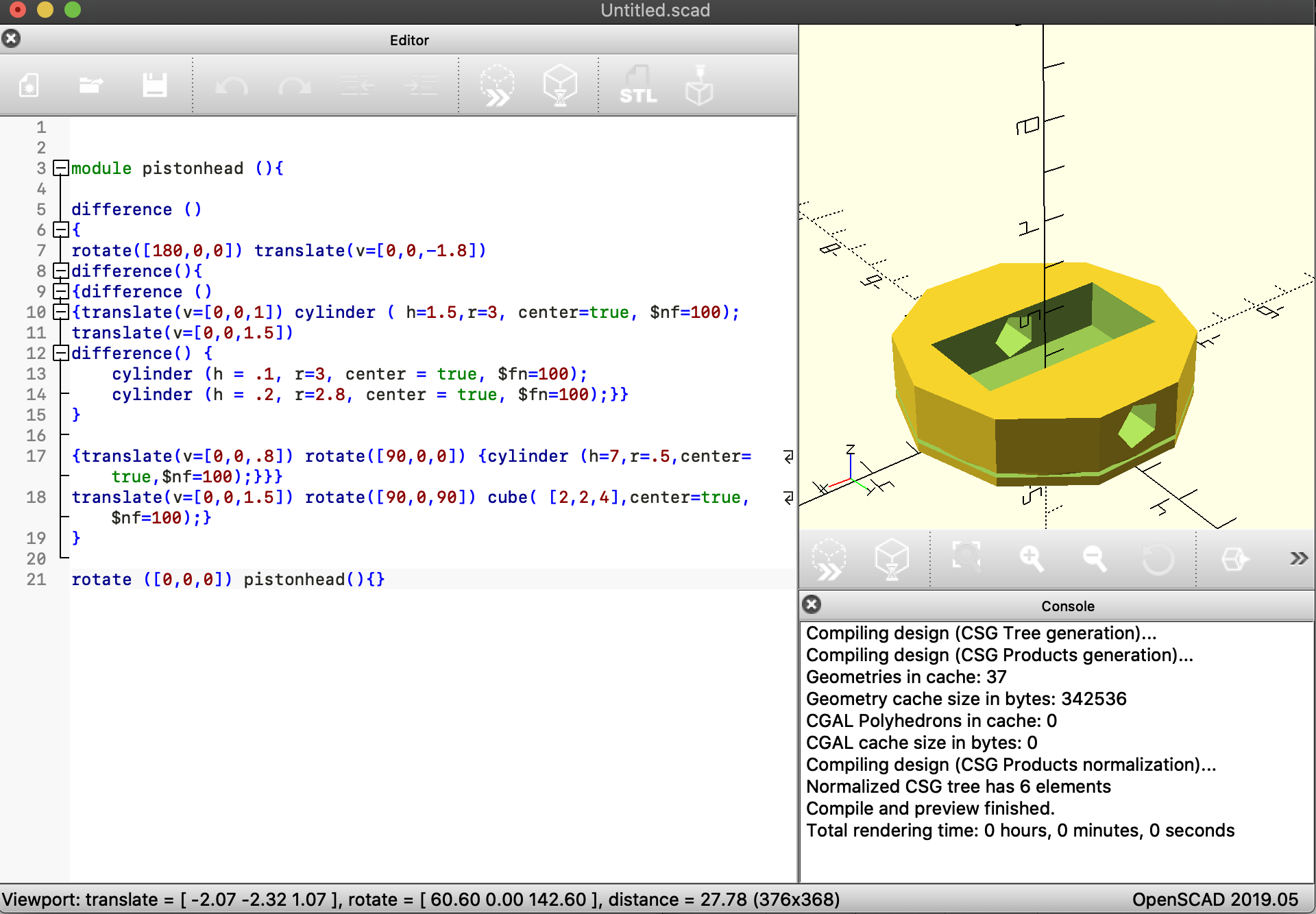Above is the piston head itself. hollowed out and has a piston ring area.

Below is the whole assembly. ignore the yellow cylinders going through the assembly that is just for me to reference.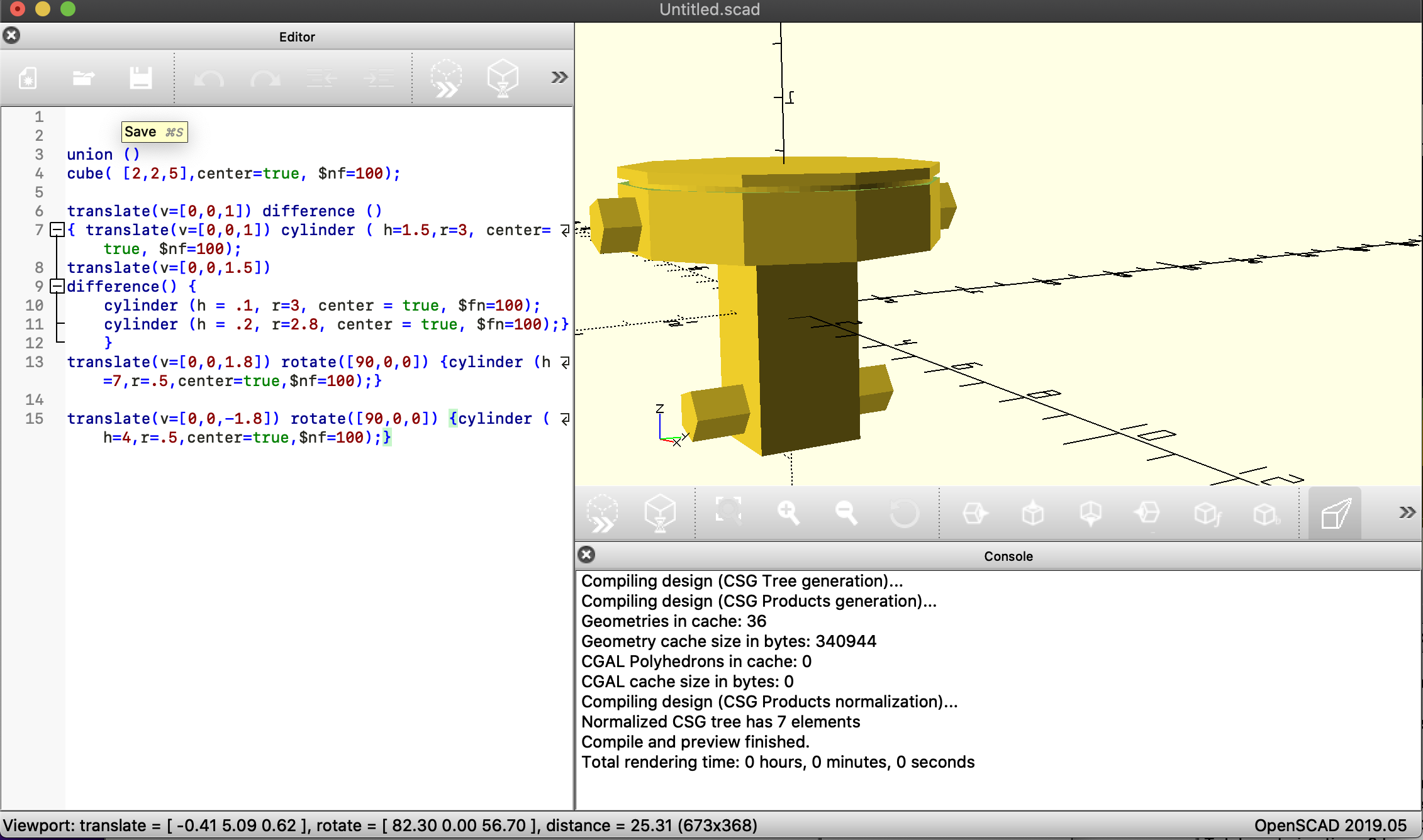So ive decided to design a piston and plan on making an engine block for the pistons to go into.

all the code is my own and is kinda basic functions but layered up. the piston head ive made into a module and will just use that in the future designs. my design process kinda just started with the piston head and moved my way down with the connecting rod. Ive included a cut out for piston rings just like a real piston needs and have hollowed out the inside of the piston head to allow for the connecting rod to move. I need to figure out how to round out the connecting rod and then make the cylinders perfectly round.

```module pistonhead (){

difference ()

{

rotate([180,0,0]) translate(v=[0,0,-1.8])

difference(){

{difference ()

{translate(v=[0,0,1]) cylinder ( h=1.5,r=3, center=true, \$nf=100);

translate(v=[0,0,1.5])

difference() {

cylinder (h = .1, r=3, center = true, \$fn=100);

cylinder (h = .2, r=2.8, center = true, \$fn=100);}}

}

{translate(v=[0,0,.8]) rotate([90,0,0]) {cylinder (h=7,r=.5,center=true,\$nf=100);}}}

translate(v=[0,0,1.5]) rotate([90,0,90]) cube( [2,2,4],center=true,\$nf=100);}

}

now this is code for the whole assembly:

```union ()

cube( [2,2,5],center=true, \$nf=100);

translate(v=[0,0,1]) difference ()

{ translate(v=[0,0,1]) cylinder ( h=1.5,r=3, center=true, \$nf=100);

translate(v=[0,0,1.5])

difference() {

cylinder (h = .1, r=3, center = true, \$fn=100);

cylinder (h = .2, r=2.8, center = true, \$fn=100);}

}

translate(v=[0,0,1.8]) rotate([90,0,0]) {cylinder (h=7,r=.5,center=true,\$nf=100);}

translate(v=[0,0,-1.8]) rotate([90,0,0]) {cylinder (h=4,r=.5,center=true,\$nf=100);}

```

for my second design ive followed a YouTube tutorial to create a simple ball bearing.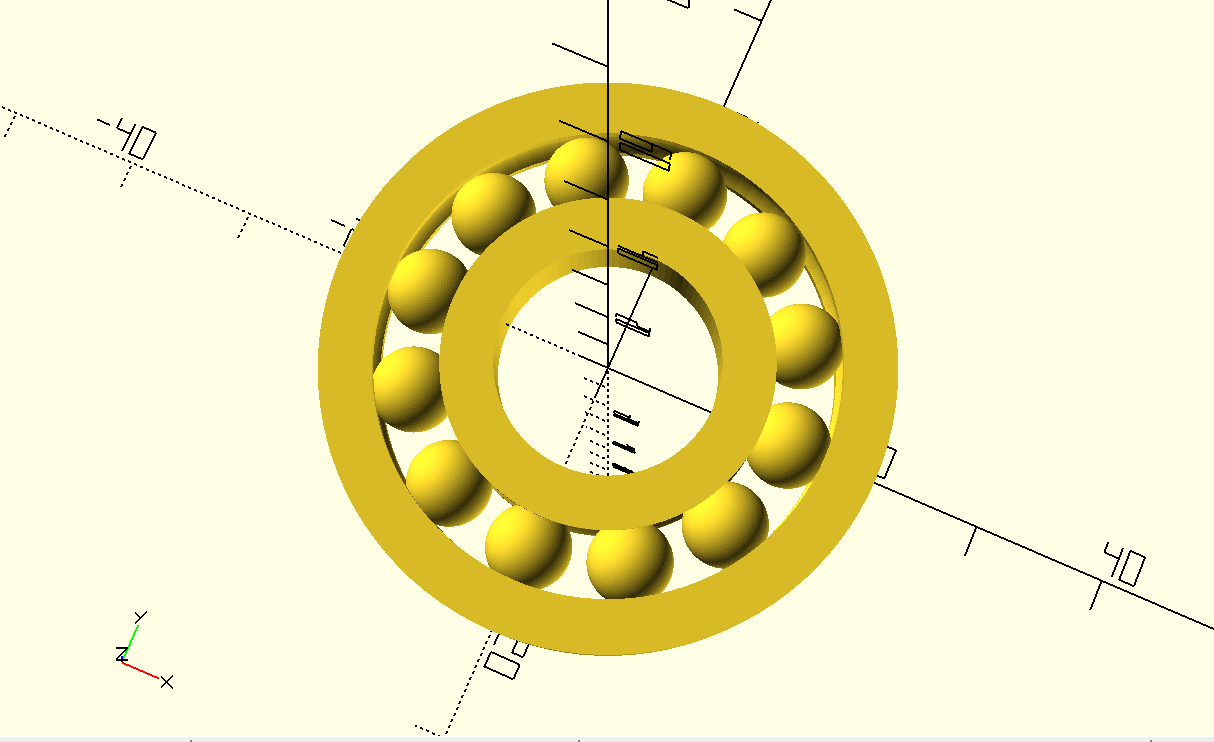in the code I utilize a couple new tools in my tool bag per say. I used the rotate and extrude command, I named variables, and I used a for loop to take an item rotate it around the axis and duplicate it around that line. I also learned some small but I think basic things I should have used in the past like \$nf= 100 to make the circles more round.

link: I need some help for some reason the copy and past is only copying my last code.. i can only hyper link the video.

further iterations maybe would see a chamfer around the out side of the bearing and them maybe put the bearing in something like a simple skate board or something like that.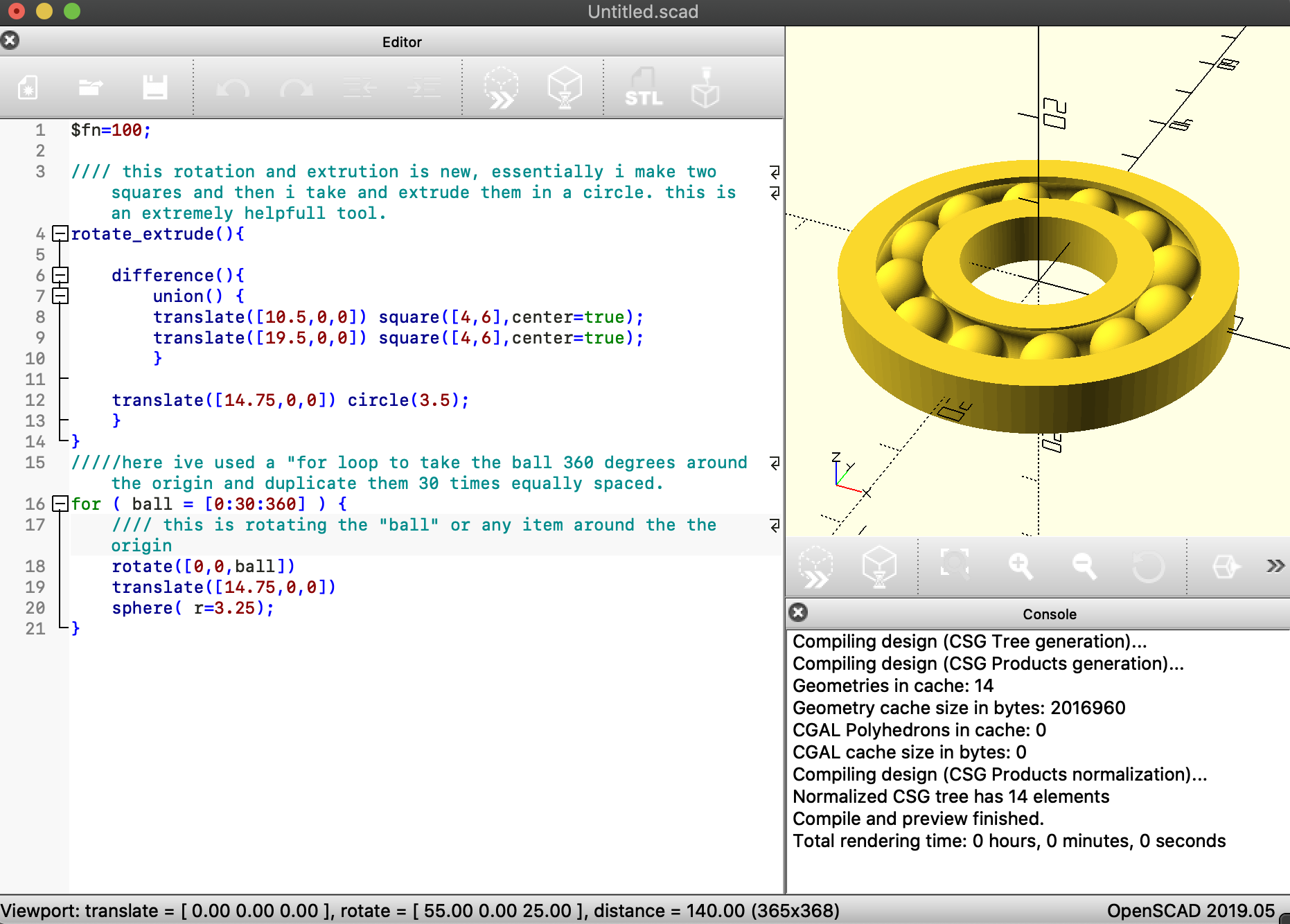code:

```\$fn=100;

//// this rotation and extrution is new, essentially i make two squares and then i take and extrude them in a circle. this is an extremely helpfull tool.
rotate_extrude(){

difference(){
union() {
translate([10.5,0,0]) square([4,6],center=true);
translate([19.5,0,0]) square([4,6],center=true);
}

translate([14.75,0,0]) circle(3.5);
}
}
/////here ive used a "for loop to take the ball 360 degrees around the origin and duplicate them 30 times equally spaced.
for ( ball = [0:30:360] ) {
//// this is rotating the "ball" or any item around the the origin
rotate([0,0,ball])
translate([14.75,0,0])
sphere( r=3.25);
}

```

## Second Openscad design **round two**

I needed to redo my second open scad design so here it is.

its a version of splunk-it where you use chopsticks to hold up marbles in the tube and each player pulls one stick out on their turn. who ever pulls the stick that drops all the balls loses.

my computer nearly shit the bed trying to render this so iterating this is going to be fun.I used the same tools I learned from my first attempt at second openscad design. rotate_extrude and for loops are the biggest newest tool I used.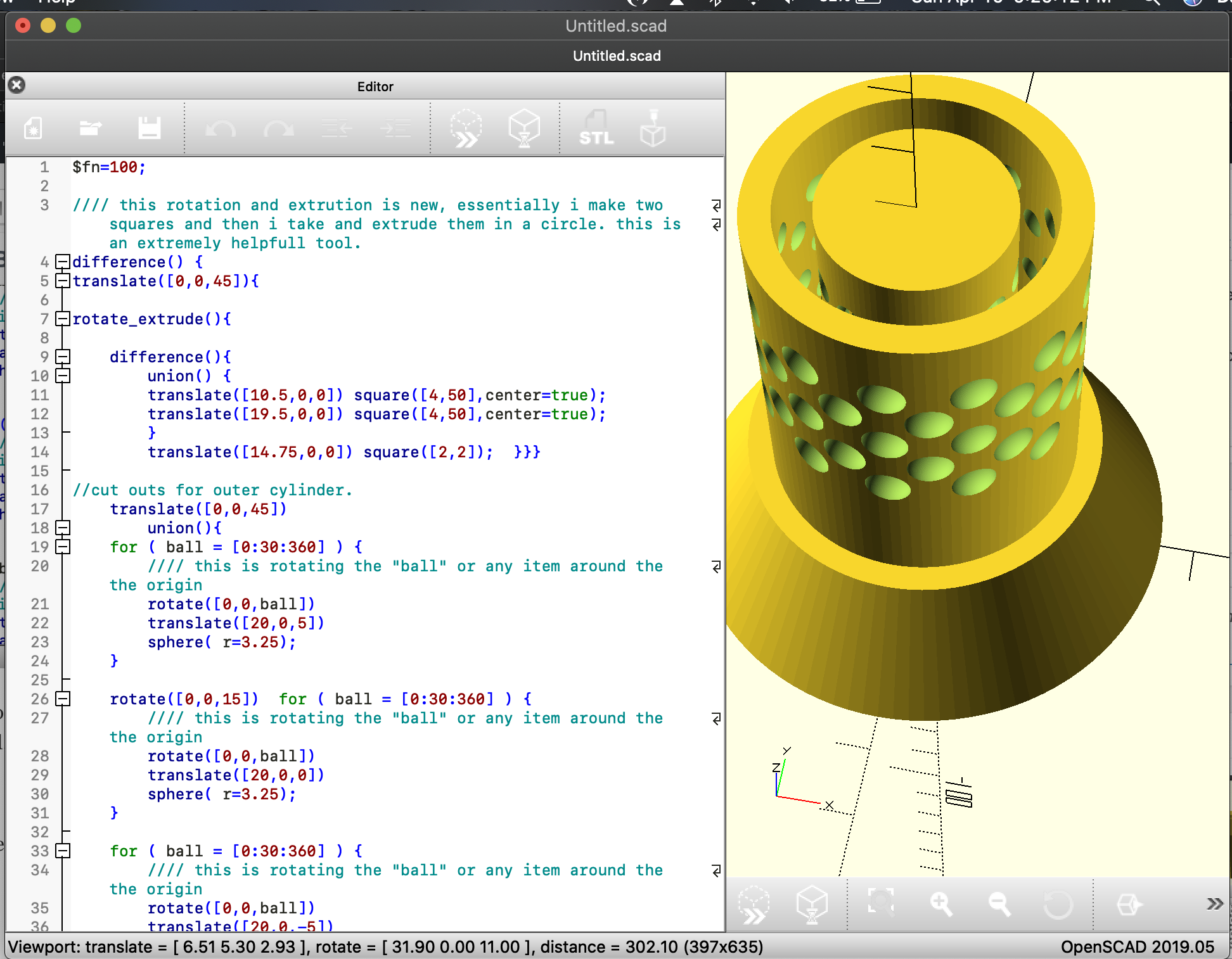perhaps for further iterations I will change the base to make it into a cup sorta thing like the real game so that it gives a nice and satisfying drop.

CODE:

\$fn=100;

//// this rotation and extrution is new, essentially i make two squares and then i take and extrude them in a circle. this is an extremely helpfull tool.

difference() {

translate([0,0,45]){

rotate_extrude(){

difference(){

union() {

translate([10.5,0,0]) square([4,50],center=true);

translate([19.5,0,0]) square([4,50],center=true);

}

translate([14.75,0,0]) square([2,2]);  }}}

//cut outs for outer cylinder.

translate([0,0,45])

union(){

for ( ball = [0:30:360] ) {

//// this is rotating the “ball” or any item around the the origin

rotate([0,0,ball])

translate([20,0,5])

sphere( r=3.25);

}

rotate([0,0,15])  for ( ball = [0:30:360] ) {

//// this is rotating the “ball” or any item around the the origin

rotate([0,0,ball])

translate([20,0,0])

sphere( r=3.25);

}

for ( ball = [0:30:360] ) {

//// this is rotating the “ball” or any item around the the origin

rotate([0,0,ball])

translate([20,0,-5])

sphere( r=3.25);

}

for ( ball = [0:30:360] ) {

//// this is rotating the “ball” or any item around the the origin

rotate([0,0,ball])

translate([20,0,15])

sphere( r=3.25);

}

rotate([0,0,15])  for ( ball = [0:30:360] ) {

//// this is rotating the “ball” or any item around the the origin

rotate([0,0,ball])

translate([20,0,10])

sphere( r=3.25);

}

// cut outs for inner cylinder

for ( ball = [0:30:360] ) {

//// this is rotating the “ball” or any item around the the origin

rotate([0,0,ball])

translate([10,0,5])

sphere( r=3.25);

}

rotate([0,0,15]) for ( ball = [0:30:360] ) {

//// this is rotating the “ball” or any item around the the origin

rotate([0,0,ball])

translate([10,0,0])

sphere( r=3.25);

}

for ( ball = [0:30:360] ) {

//// this is rotating the “ball” or any item around the the origin

rotate([0,0,ball])

translate([10,0,-5])

sphere( r=3.25);

}

for ( ball = [0:30:360] ) {

//// this is rotating the “ball” or any item around the the origin

rotate([0,0,ball])

translate([10,0,15])

sphere( r=3.25);

}

rotate([0,0,15])for ( ball = [0:30:360] ) {

//// this is rotating the “ball” or any item around the the origin

rotate([0,0,ball])

translate([10,0,10])

sphere( r=3.25);

}

}}

cylinder(h = 20, r1 = 35, r2 = 25);

translate([0,0,65]) cylinder(h=5,r=10.5);

As I talked about previously Im adding a cup to the Botton so the marbles can drop to the bottom and make a really nice noice when it all comes tumbling down. I also added a storage cup to the top with a lid so the whole game is pretty portable and packages nicely.
this image is with out the holes so I can save my computer from cooking itself while trying to render.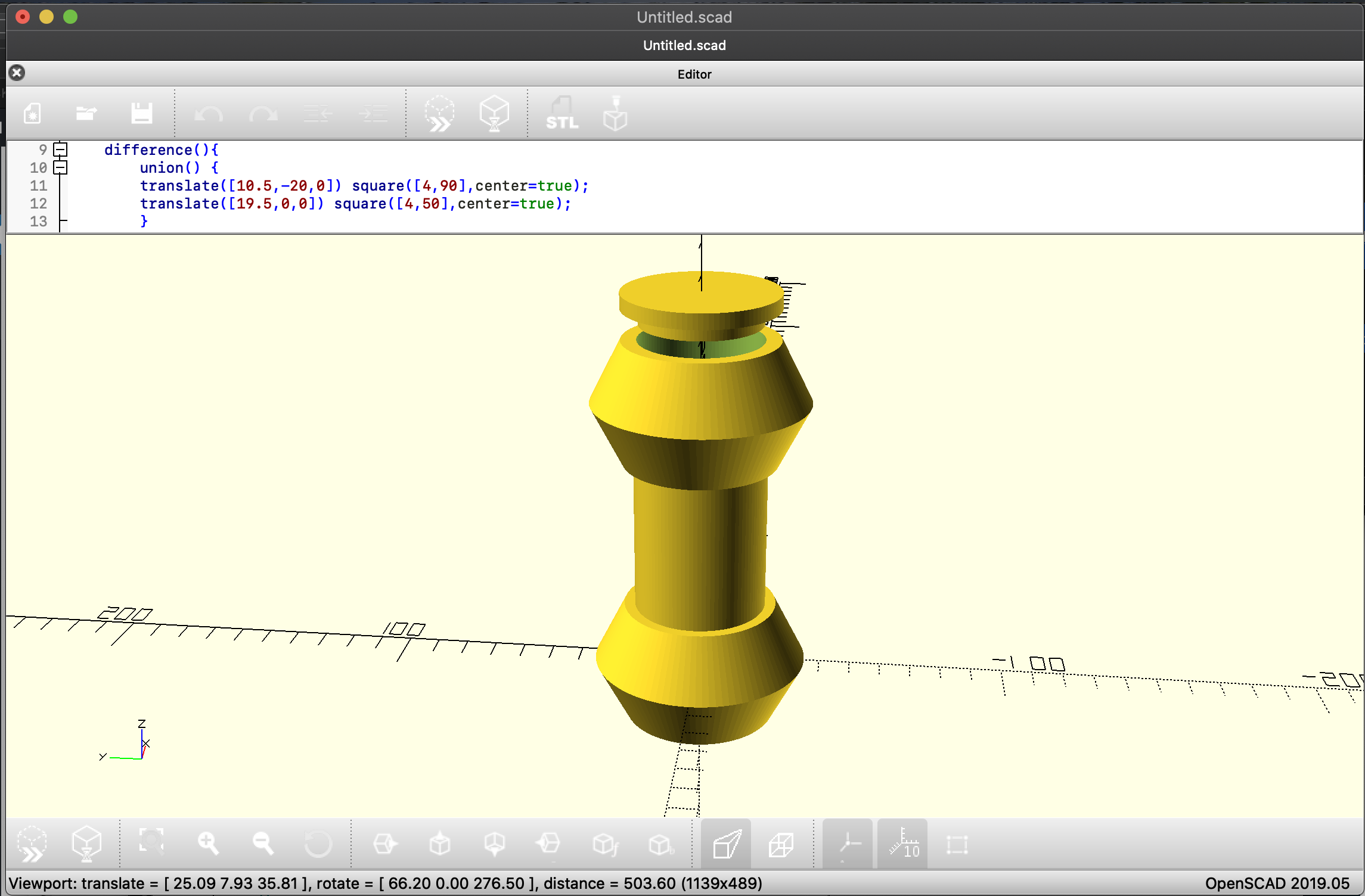I just coded the top and bottom containers. this posed a problem when I hollowed out the bottom container cause the central tube didn’t have any support so I needed to extend that tube and push it to the bottom so it is one piece with the bottom of the container. I slo made a lid and needed to make the spacing just right. this wasn’t super difficult.
here is a photo go the complete product with the holes. RIP my computer 2017-2020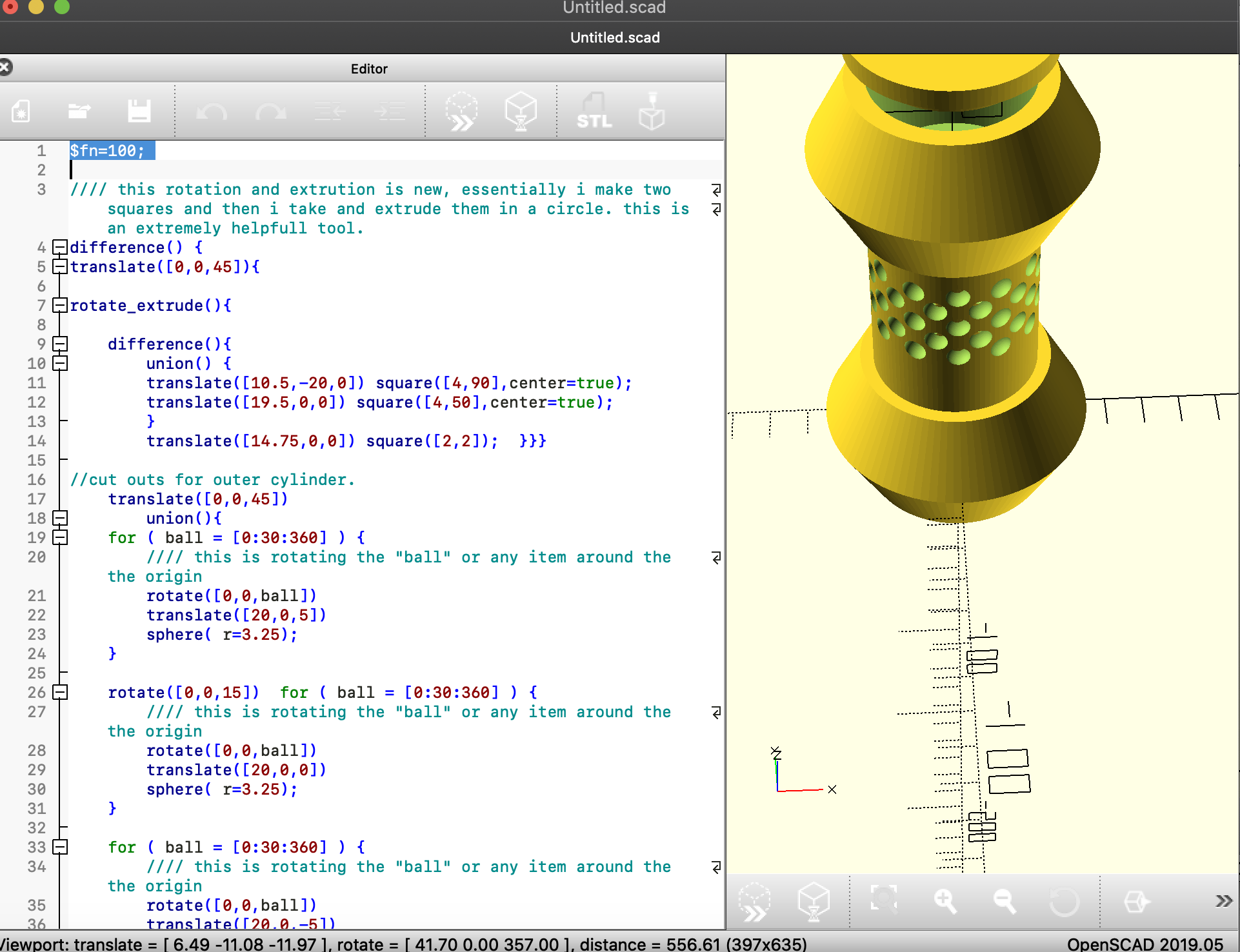CODE:
```\$fn=100;

//// this rotation and extrution is new, essentially i make two squares and then i take and extrude them in a circle. this is an extremely helpfull tool.
difference() {
translate([0,0,45]){

rotate_extrude(){

difference(){
union() {
translate([10.5,-20,0]) square([4,90],center=true);
translate([19.5,0,0]) square([4,50],center=true);
}
translate([14.75,0,0]) square([2,2]); }}}

//cut outs for outer cylinder.
translate([0,0,45])
union(){
for ( ball = [0:30:360] ) {
//// this is rotating the "ball" or any item around the the origin
rotate([0,0,ball])
translate([20,0,5])
sphere( r=3.25);
}

rotate([0,0,15]) for ( ball = [0:30:360] ) {
//// this is rotating the "ball" or any item around the the origin
rotate([0,0,ball])
translate([20,0,0])
sphere( r=3.25);
}

for ( ball = [0:30:360] ) {
//// this is rotating the "ball" or any item around the the origin
rotate([0,0,ball])
translate([20,0,-5])
sphere( r=3.25);
}
for ( ball = [0:30:360] ) {
//// this is rotating the "ball" or any item around the the origin
rotate([0,0,ball])
translate([20,0,15])
sphere( r=3.25);
}
rotate([0,0,15]) for ( ball = [0:30:360] ) {
//// this is rotating the "ball" or any item around the the origin
rotate([0,0,ball])
translate([20,0,10])
sphere( r=3.25);
}

// cut outs for inner cylinder
for ( ball = [0:30:360] ) {
//// this is rotating the "ball" or any item around the the origin
rotate([0,0,ball])
translate([10,0,5])
sphere( r=3.25);
}

rotate([0,0,15]) for ( ball = [0:30:360] ) {
//// this is rotating the "ball" or any item around the the origin
rotate([0,0,ball])
translate([10,0,0])
sphere( r=3.25);
}

for ( ball = [0:30:360] ) {
//// this is rotating the "ball" or any item around the the origin
rotate([0,0,ball])
translate([10,0,-5])
sphere( r=3.25);
}
for ( ball = [0:30:360] ) {
//// this is rotating the "ball" or any item around the the origin
rotate([0,0,ball])
translate([10,0,15])
sphere( r=3.25);
}
rotate([0,0,15])for ( ball = [0:30:360] ) {
//// this is rotating the "ball" or any item around the the origin
rotate([0,0,ball])
translate([10,0,10])
sphere( r=3.25);
}

}}
//bottom bottle that is the marble catch
difference (){
union(){
cylinder (h = 20, r1 = 35, r2 = 25);
rotate ([0,180,0]) cylinder (h = 20, r1 = 35, r2 = 25);}

translate([0,0,10]) cylinder (h=40,r=20,center=true);}

//top bobble that is also a marble storage bin
translate([0,0,90])
difference (){
union(){
cylinder (h = 20, r1 = 35, r2 = 25);
rotate ([0,180,0]) cylinder (h = 20, r1 = 35, r2 = 25);}

translate([0,0,10]) cylinder (h=40,r=20,center=true);}

//cap
translate([0,0,100]) union(){
translate([0,0,20]) cylinder (h=5,r=25);
translate([0,0,15]) cylinder (h=5,r=19.5);}```

so for my randomness I mixed up how many and where the holes for the chopsticks are. both on the inner and outer tubes. I also added some randomness to how far down the drop is to the bottom catch can. this needs some work and further iterations to get just right but maybe that will be for my final show case. this is nice because It can change the experience of the game. it can make a super high tower that is easy to knock over in which case you lose like jenga and it also changes the auditory experience of the game so when the balls have a long way to drop they make a louder noise or a softer noise etc.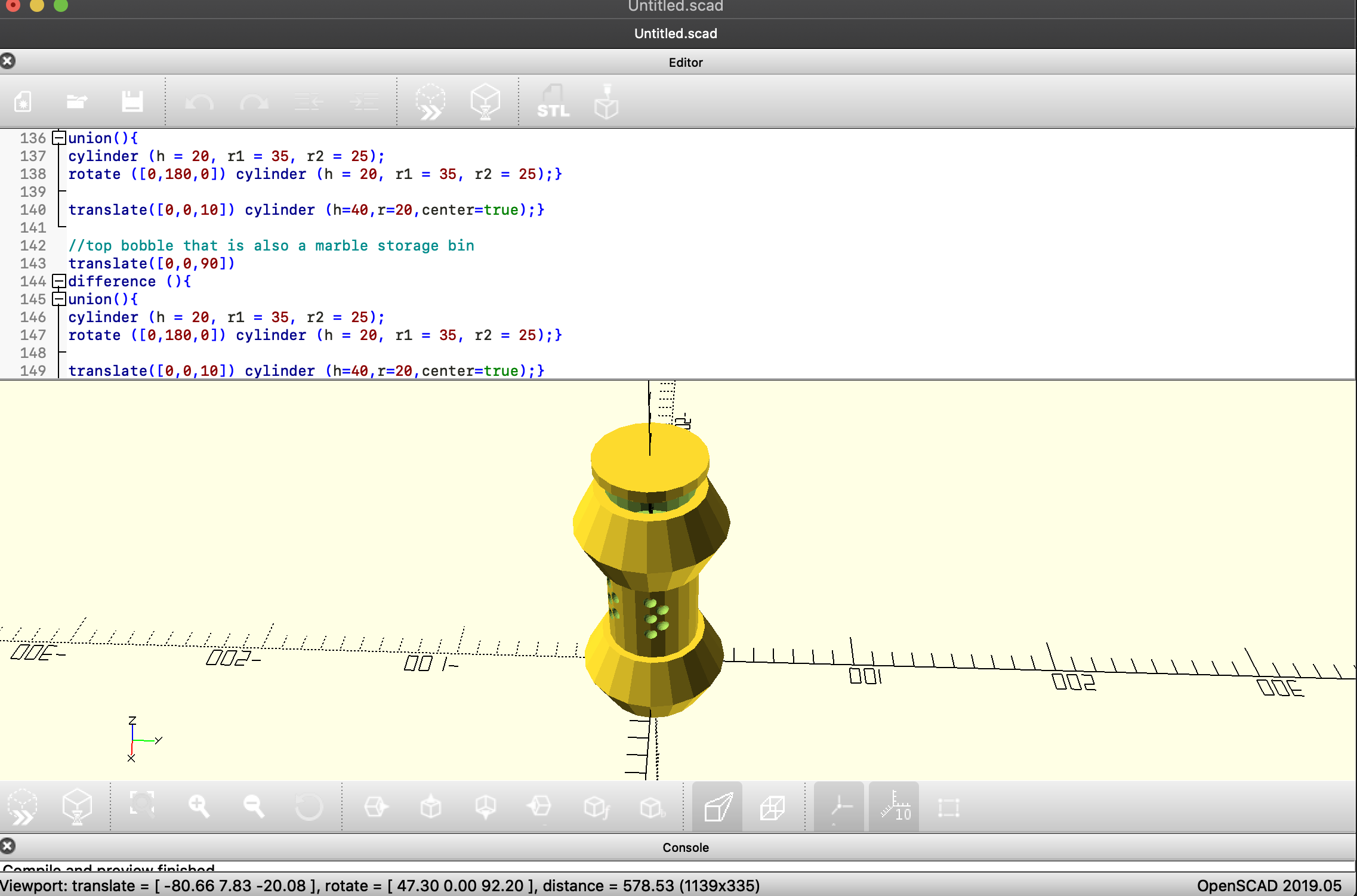I added two photos to see how it changeshere is my code:

```// LET OPENSCAD CHOOSE A RANDOM SEED random_seed = floor(rands(1,9999999,1));
// OR TYPE IN A SEED MANUALLY
// Overrides the slider seed unless set to 0 custom_seed = 0;
// set the random seed seed = (custom_seed==0) ? random_seed : custom_seed;
// a list of 30 random real numbers in [0,10] based on the chosen seed
// to use these in the code type random, random, random, and so on maxSteps=30; random = rands(0,10,maxSteps,seed);
///////////////////////////////////////////////////////////////////
// DISPLAY THE SEED
// Show random seed? (it won't print even if you say yes) show_seed = "yes";
//[yes:Yes,no:No]
// Create a string to output the random seed
// thank you laird for showing me how to do this with your code
// from http://www.thingiverse.com/thing:188481 labelString=str(floor(seed/1000000)%10, floor(seed/100000)%10,
floor(seed/10000)%10, floor(seed/1000)%10,
floor(seed/100)%10, floor(seed/10)%10,
floor(seed/1)%10);
// Display the random seed in the render (F5 only)
if(show_seed=="yes"){
translate([0,-30,0])
color("gray")
%linear_extrude(height=1)
text(labelString,size=4,halign="center"); }
// Also output the random seed and the resulting list of random numbers in the log echo(labelString); echo(random);
///////////////////////////////////////////////////////////////////
// OK NOW MAKE SOMETHING! \$fn=20;
//// this rotation and extrution is new, essentially i make two squares and then i take and extrude them in a circle.
this is an extremely helpfull tool.
difference() { translate([0,0,45]){ rotate_extrude(){ difference(){ union()
{ translate([10.5,-20,0]) square([4,90],center=true); translate([19.5,0,0]) square([4,50],center=true); }
translate([14.75,0,0]) square([2,2]); }}} //cut outs for outer cylinder. translate([0,0,45])
union(){ for ( ball = [0:30*random:360] ) {
//// this is rotating the “ball” or any item around the the origin
rotate([0,0,ball]) translate([20,0,5]) sphere( r=3.25); }
rotate([0,0,15]) for ( ball = [0:30*random:360] ) {
//// this is rotating the “ball” or any item around the the origin
rotate([0,0,ball]) translate([20,0,0]) sphere( r=3.25); }
for ( ball = [0:30*random:360] ) {
//// this is rotating the “ball” or any item around the the origin
rotate([0,0,ball]) translate([20,0,-5]) sphere( r=3.25); }
for ( ball = [0:30*random:360] ) {
//// this is rotating the “ball” or any item around the the origin
rotate([0,0,ball]) translate([20,0,15]) sphere( r=3.25); }
rotate([0,0,15]) for ( ball = [0:30*random:360] ) {
//// this is rotating the “ball” or any item around the the origin
rotate([0,0,ball]) translate([20,0,10]) sphere( r=3.25); }
// cut outs for inner cylinder for ( ball = [0:30*random:360] ) {
//// this is rotating the “ball” or any item around the the origin
rotate([0,0,ball]) translate([10,0,5]) sphere( r=3.25); }
rotate([0,0,15]) for ( ball = [0:30*random:360] ) {
//// this is rotating the “ball” or any item around the the origin
rotate([0,0,ball]) translate([10,0,0]) sphere( r=3.25); }
for ( ball = [0:30*random:360] ) {
//// this is rotating the “ball” or any item around the the origin
rotate([0,0,ball]) translate([10,0,-5]) sphere( r=3.25); }
for ( ball = [0:30*random:360] ) {
//// this is rotating the “ball” or any item around the the origin
rotate([0,0,ball]) translate([10,0,15]) sphere( r=3.25); }
rotate([0,0,15])for ( ball = [0:30*random:360] ) {
//// this is rotating the “ball” or any item around the the origin
rotate([0,0,ball]) translate([10,0,10]) sphere( r=3.25); } }}
//bottom bottle that is the marble catch
translate([0,0,1*random+2])
difference (){ union(){ cylinder (h = 20, r1 = 35, r2 = 25);
rotate ([0,180,0]) cylinder (h = 20, r1 = 35, r2 = 25);} translate([0,0,10]) cylinder (h=40,r=20,center=true);}
//top bobble that is also a marble storage bin
translate([0,0,90]) difference (){
union(){ cylinder (h = 20, r1 = 35, r2 = 25);
rotate ([0,180,0]) cylinder (h = 20, r1 = 35, r2 = 25);}
translate([0,0,10]) cylinder (h=40,r=20,center=true);}
//cap
translate([0,0,100])
union(){ translate([0,0,20]) cylinder (h=5,r=25); translate([0,0,15]) cylinder (h=5,r=19.5);}```

## Final project and randomness.

largely my code stayed the same but I tried to figure out how to make the bottom marble catch bin move up and down with the randomness but had to abandon that idea because I couldn’t figure out how to set boundaries and I didn’t want the catch bin to become detached out of nowhere.
I also forgot to add the randomness to the inside holes so I did that.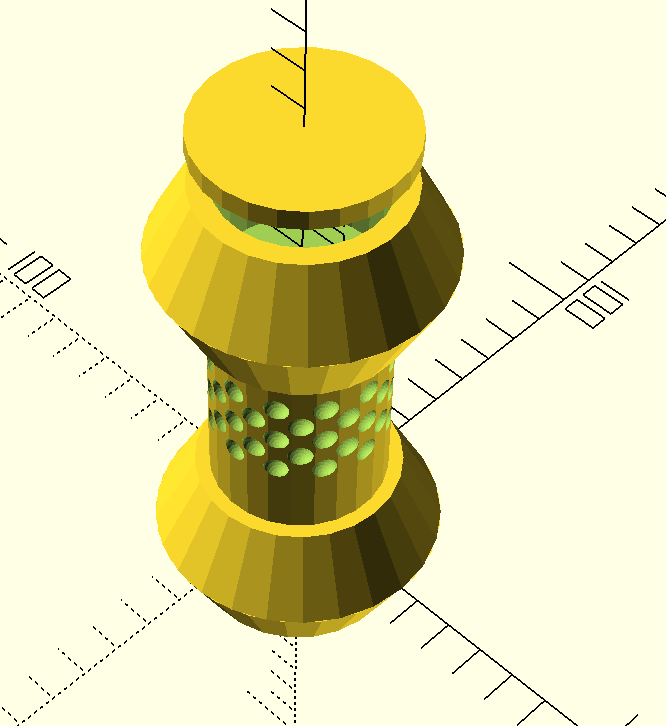code:

// LET OPENSCAD CHOOSE A RANDOM SEED
random_seed = floor(rands(1,9999999,1));

// OR TYPE IN A SEED MANUALLY
// Overrides the slider seed unless set to 0
custom_seed = 0;

// set the random seed
seed = (custom_seed==0) ? random_seed : custom_seed;

// a list of 30 random real numbers in [0,10] based on the chosen seed
// to use these in the code type random, random, random, and so on
maxSteps=30;
random = rands(0,10,maxSteps,seed);

///////////////////////////////////////////////////////////////////
// DISPLAY THE SEED

// Show random seed? (it won’t print even if you say yes)
show_seed = “yes”; //[yes:Yes,no:No]

// Create a string to output the random seed
// thank you laird for showing me how to do this with your code
// from http://www.thingiverse.com/thing:188481
labelString=str(floor(seed/1000000)%10, floor(seed/100000)%10,
floor(seed/10000)%10, floor(seed/1000)%10,
floor(seed/100)%10, floor(seed/10)%10,
floor(seed/1)%10);

// Display the random seed in the render (F5 only)
if(show_seed==”yes”){
translate([0,-30,0])
color(“gray”)
%linear_extrude(height=1)
text(labelString,size=4,halign=”center”);
}

// Also output the random seed and the resulting list of random numbers in the log
echo(labelString);
echo(random);

///////////////////////////////////////////////////////////////////
// OK NOW MAKE SOMETHING!

\$fn=20;

//// this rotation and extrution is new, essentially i make two squares and then i take and extrude them in a circle. this is an extremely helpfull tool.
difference() {
translate([0,0,45]){

rotate_extrude(){

difference(){
union() {
translate([10.5,-20,0]) square([4,90],center=true);
translate([19.5,0,0]) square([4,50],center=true);
}
translate([14.75,0,0]) square([2,2]); }}}

//cut outs for outer cylinder.
translate([0,0,45])
union(){
for ( ball = [0:30*random:360] ) {
//// this is rotating the “ball” or any item around the the origin
rotate([0,0,ball])
translate([20,0,5])
sphere( r=3.25);
}

rotate([0,0,15]) for ( ball = [0:30*random:360] ) {
//// this is rotating the “ball” or any item around the the origin
rotate([0,0,ball])
translate([20,0,0])
sphere( r=3.25);
}

for ( ball = [0:30*random:36